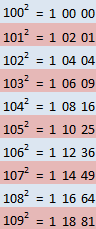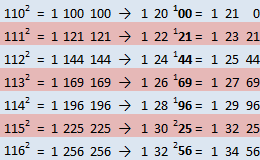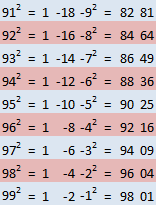Squaring a number close to 100

This is anoter squaring trick ,this time for finding the square of numbers that are close to 100.

Case 1 (Number between 100-109)
Let us use 106 for example

The answer will be of this form
1abcd

To find what the rest of the numbers are do the following
a)    Find how much bigger than 100 the number is
106-100= 6

b)    Double this difference 6×2=12 and put this to positions ab
112cd

c)    Square this difference 6×6=36 and put this to positions cd
11236

Bellow you can see how it works for numbers between 100-109Case 2 (Number greater or equal to 110)
Let us use 112 for example

To find what the rest of the numbers are do the following
a)    Find how much bigger than 100 the number is
112-100= 12

b)    Double this difference 12×2=24
124cd

c)    Square this difference 12×12=144 and put this to positions cd
124144

d)    Since we used 3 instead of 2 numbers for positions cd, we need to treat the extra number as a carry.
124144 -> 124144-> 12544
12544 is the answer

A few examplesCase 3 (Number under 100)

As the number is under 100 the form will be like that

abcd

To find what the rest of the numbers are do the following
a)    Find how much bigger than 100 the number is
97-100= -3

b)    Double this difference
-3×2=-6
Subtract this number from 100 and put it to positions ab
100+(-6)=94
94cd

c)    Square this difference (-3)x(-3)=09  and put this to positions cd
9409

A few examples